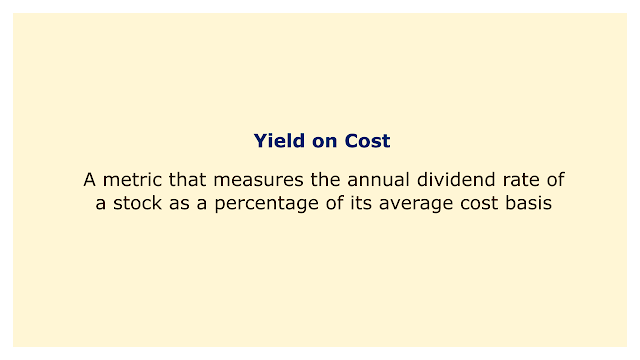# Yield on CostImage: Moneybestpal.com

### The yield on cost is a metric that gauges a stock's yearly dividend yield as a proportion of its mean cost basis. By dividing the average cost per share by the annual dividend per share, it is computed.

Let's say, for instance, that you paid \$10 for 100 shares of the XYZ corporation and received a \$0.50 dividend per share. Your yield on cost would be:

Yield on cost = (\$0.50 x 100) / (\$10 x 100) = 0.05 or 5%

Let's say you received another \$0.50 dividend per share and purchased another 100 shares of the XYZ firm for \$20 each. Your average cost basis would be:

Average cost basis = [(\$10 x 100) + (\$20 x 100)] / 200 = \$15

Your yield on cost would be:

Yield on cost = (\$0.50 x 200) / (\$15 x 200) = 0.0333 or 3.33%

As can be seen, your yield on cost declines as your average cost basis rises. Hence, if the stock price increases, you will receive a lower return on your investment.

The market value of your investment, however, is not reflected in yield on cost. Only the amount of revenue you are making from your purchase price is displayed. The present yield or dividend yield should be considered if you want to know how much your investment is currently worth.

The annual dividend per share is divided by the share's market price at the time of calculation to get the current yield. For example, if XYZ company is trading at \$25 per share and pays a \$0.50 dividend per share, its current yield would be:

Current yield = (\$0.50 x 100) / (\$25 x 100) = 0.02 or 2%

The dividend yield is comparable to the current yield, but instead of using the yearly dividend, it uses the most recent dividend payment. For example, if XYZ company pays a quarterly dividend of \$0.125 per share and is trading at \$25 per share, its dividend yield would be:

Dividend yield = (\$0.125 x 100) / (\$25 x 100) = 0.005 or 0.5%

Depending on your investment's current market value, both the current yield and dividend yield show you how much income you are receiving. If you want to measure the performance of your portfolio or compare several equities, they are more important than the yield on cost.

But, if you want to gauge how much your dividend income has increased in comparison to your initial investment over time, yield on cost can be helpful. Based on a stock's potential for dividend growth, it can also assist you in determining whether to hold or sell it.

For instance, regardless of changes in the stock price, XYZ Company's yield on cost will increase by 10% if its dividend is increased by 10% each year. As long as you own the stock, this implies that you will continue to receive a higher return on your investment each year.

Contrarily, independent of changes in the stock price, if XYZ Company reduces its dividend by 50%, the yield on cost will also fall by 50%. Hence, unless you sell the stock, you will receive a lower return on your investment each year.
Tags